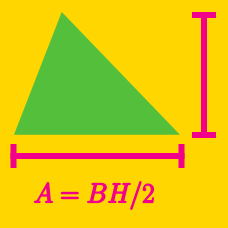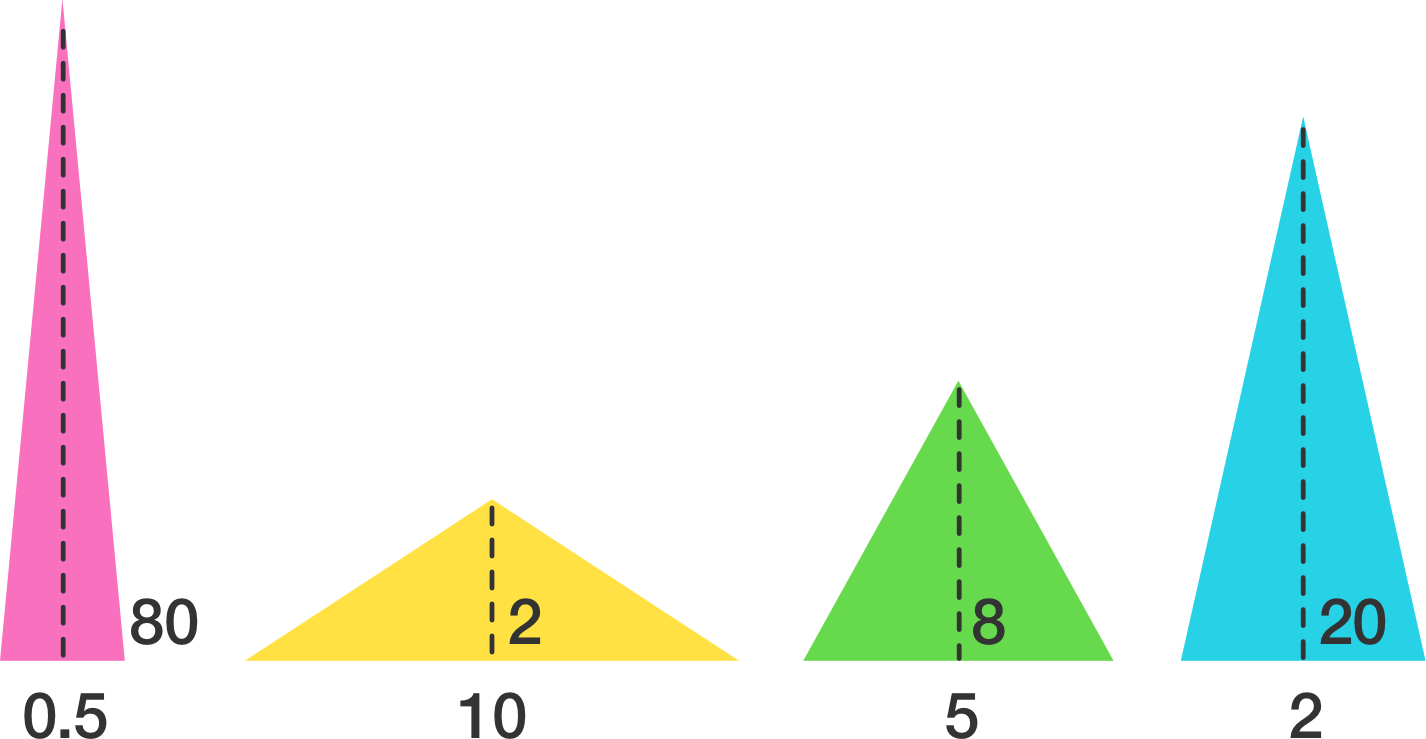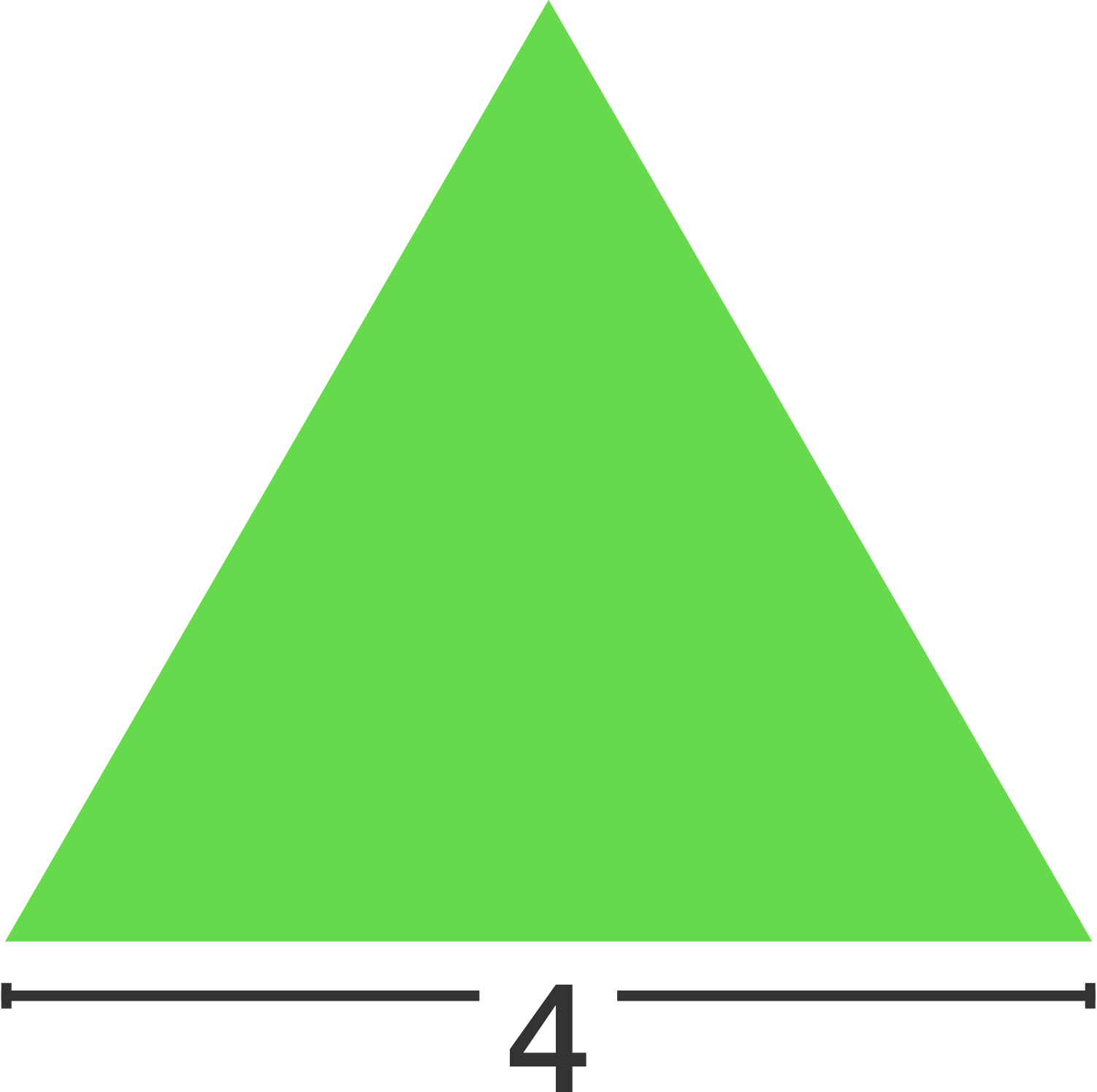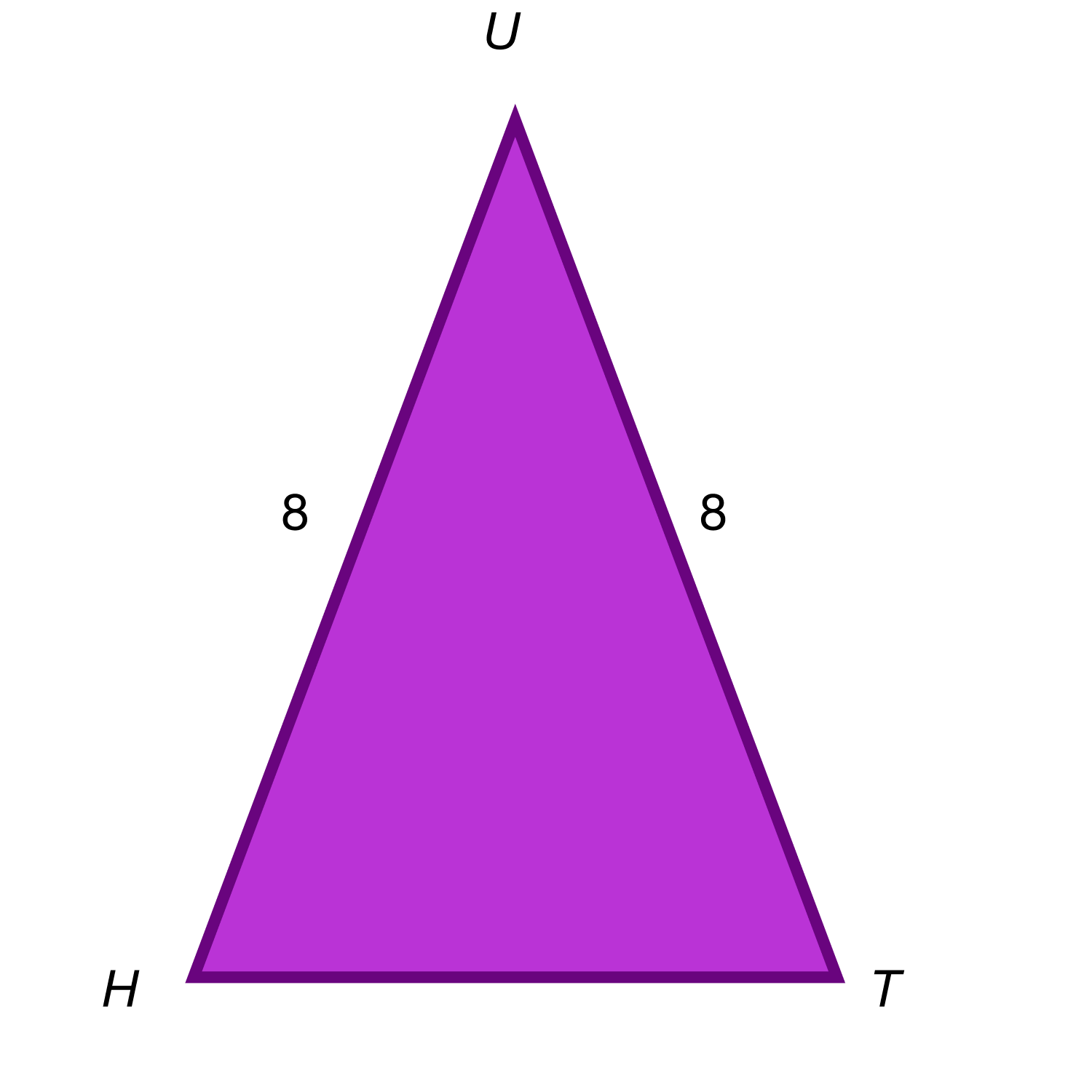Geometry

# Area of Triangles WarmupA triangle has an area of 20 square units. Which set of values could NOT represent the base and height?

Which has the greater area:

A: A square with side lengths of $x.$

B. A triangle with base length $x$ that is twice as tall as it is wide.

Shayna draws triangle $P$ with a base and a height greater than one. She triples the base length and halves the height to get triangle $Q.$ Which triangle has greater area?An equilateral triangle has a base length of 4. What is its area?One way to find the area of a triangle is to use the Sine Rule: Area = $\frac{1}{2}ab \sin{C}.$ In the formula, $a$ and $b$ are the adjacent sides to any angle angle $C$ of the triangle.

If $\text {sin }\angle HUT$ is $\frac{1}{2},$ what is the area of $\triangle HUT?$

×